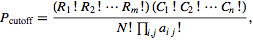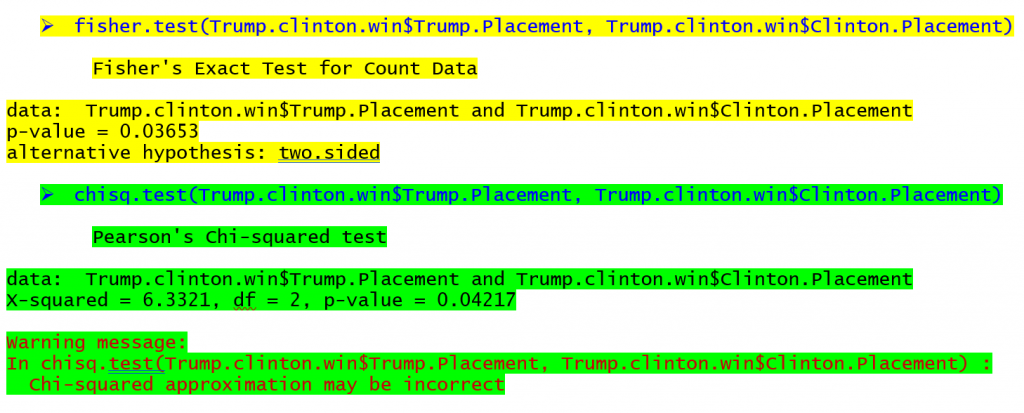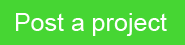This post is authored by Paul Ricci, a Kolabtree expert. It originally appeared in his column on Data Driven Journalism.

This article outlines how Fisher’s exact test can be used for small sample contingency tables. A common problem in data analysis is how to determine if there is a statistical relationship between two categorical variables such as gender, race, or the share of the vote for two candidates in an election.  The simplest way to visualize the relationship is to represent the counts for each combination of two variables in a contingency table with the rows representing the levels of one variable and the columns representing the levels of the other variable.  The most commonly used statistical test for an association between the row and column variables is the chi-square (χ2) test.  The example in the table below is given to illustrate the test.

 Democrat Winner (% of column) Total Clinton Win Sanders win Trump 1st 25 (86%) 12 (55%) 37 Trump 2nd 3 (11%) 8 (36%) 11 Trump 3rd 1 (3%) 2 (9%) 3 Total 29 (100%) 22 (100%) 51

The columns in the above table shows the primary states won by Hillary Clinton and by Bernie Sanders on the Democratic side and Donald Trump placed in the same primary states on the Republican side. The total number of states in the table is 51 because the District of Columbia is included. The column percent’s show that Trump won 86% of the primary states that Clinton won while he won 55% of the states that Sanders won.

The chi-square test is based on calculating expected values for each cell in the table. For example, the expected value (the value for the cell which one would expect to see if there were no relationship mong the variables) for the cell for states where Trump finished third on the Republican side and for states where Bernie Sanders won on the Democratic side would be computed by multiplying the row total for where Trump placed third (3) by the column total for states where Sanders won (22). This product is then divided by the total number of observations for (51). The formula for the expected value is given by:That means that for this cell a value of 1.29 would be expected if the primary states where Trump finished third and Sanders won were completely independent of each other.  The observed value for this cell is 2 suggesting a higher count for this cell than would be expected.  Expected values would be computed for each cell in the table and the difference between the observed and expected values for each cell is computed, squared, divided by the expected value, and summed across the cells in the table according to the formula:If the value for the chi-square exceeds the chi-square critical value for a given degree of freedom (found by multiplying the number of rows minus one and the number of columns minus one) and p-value, it is concluded that there is an association between the variables.

There is a problem with the chi-square test.  It is an approximation of the distribution of counts in contingency tables.  If more than 20% of the cells in the table have an expected value of less than five, the chi-square approximation does not work to test the hypothesis of an association between the row variable and the column variable (as is the case in the table below).  Both variables in the table are categorical.  The major statistical packages will alert the user if this assumption is violated.  Violating the assumption causes the observed p-value to be incorrect and can lead to incorrect conclusions being made regarding the presence or absence of an association.  There is an exact alternative to the chi-square test called Fisher’s exact test.

Fisher’s exact test is based on the hypergeometric probability distribution.Here the Ri! are the factorials of the row totals (5!=5*4*3*2*1), Ci! are the factorials of the individual column totals, N! is the factorial of the table total and the aij! are the factorials for the individual cell values.  The Πij is the product coefficient of the individual cell values.  Such a formula is even more computationally intensive than the chi-square test, especially for tables with many rows and columns.  This is why the chi-square test was favored in the past because it took too much memory for computers to run.  These days it is less of an issue for computers to run the Fisher’s exact test and it is easy to run in the major statistical packages (R, SAS, SPSS, STATA, etc.).

The commands to conduct Fisher’s exact test and the chi-square test in R (a free program) can be seen below for the table at the top of the article with the corresponding output (yellow for Fisher’s exact test, green for the chi-square test).The output for the Fisher’s exact test shows that there is a probability of 0.03653 of observing these table frequencies when there is no association between the rows and columns.  The chi-square test output shows a probability of 0.04217 for a relationship in the same table.  If we were using the .05 p value as the criteria for significance we would find a relationship for both tests in this case though the p-values differ.  States which Hillary Clinton won in the primary season were more likely to be won by Donald Trump while states where Bernie Sanders won were more likely to have Trump finish 2nd or 3rd In tables with even smaller sample sizes the difference between the p-values may be even greater leading to radically different conclusions.

As a warning the p-value should not be used as an indicator of the strength of the association between categorical variables.  Either the test is significant or not.  The p-value is sensitive to sample size.  Often the odds ratio is used to estimate the effect size but R only computes it in the fisher.test function for tables with 2 columns and 2 rows.

Fisher’s exact test provides a criterion for deciding whether the differences in observed percentages between two categorical variables in a sample are significant or just due to random noise in the data.  In the above example, the 86% of primary states won by Clinton and Trump are significantly different from the 55% of primary won by Sanders and Trump.  Journalists should always be careful about making these judgments by just looking at observed percentages or counts because of the subjectivity of such decisions.  Subjective decisions can be further clouded by ones preconceived notions about the issues related to the data.

Kolabtree helps businesses worldwide hire experts on demand. Our freelancers have helped companies publish research papers, develop products, analyze data, and more. It only takes a minute to tell us what you need done and get quotes from experts for free.Share.